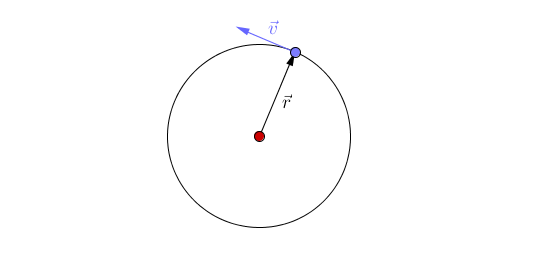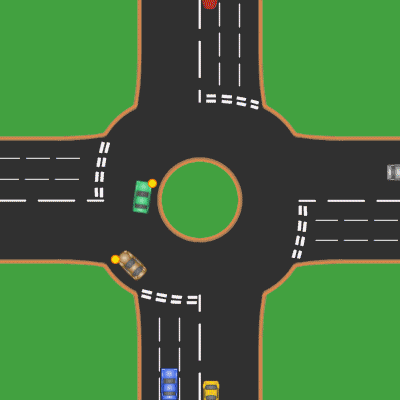# Acceleration

## Key Stage 3

### Meaning

Acceleration is an increase in speed.

Noun: Acceleration
Verb: To accelerate

The opposite of acceleration is deceleration which is to slow down.
Acceleration happens when an object experiences Unbalanced Forces.

### Acceleration Equation

$$a = {\frac{v-u}{t}}$$

Where:

a = acceleration
v = final speed
u = initial speed
t = time

### Example Calculations

 A person starts at rest and accelerates to a speed of 8m/s in 0.8 seconds. Calculate the acceleration of the person. A racing car travels comes around a corner at a speed of 20m/s and in 1.5 seconds accelerates to a speed of 80m/s. Calculate the acceleration of the racing car. final speed = 8m/s initial speed = 0m/s time = 0.8s $$a = {\frac{v-u}{t}}$$ $$a = {\frac{8-0}{0.8}}$$ $$a = {\frac{8}{0.8}}$$ $$a = 10m/s/s$$ final speed = 80m/s initial speed = 20m/s time = 1.5s $$a = {\frac{v-u}{t}}$$ $$a = {\frac{80-20}{1.5}}$$ $$a = {\frac{60}{1.5}}$$ $$a = 40m/s/s$$ A horse begins trotting at 3.0m/s and accelerates to canter at 11m/s in 2.0 seconds. Calculate the acceleration of the horse. A space probe is travelling at 18,000m/s and uses a thruster for 250 seconds to slow down to 6,000m/s. Calculate the acceleration of the space probe. final speed = 11m/s initial speed = 3.0m/s time = 2.0s $$a = {\frac{v-u}{t}}$$ $$a = {\frac{11-3.0}{2.0}}$$ $$a = {\frac{8.0}{2.0}}$$ $$a = 4m/s/s$$ final speed = 18,000m/s initial speed = 6,000m/s time = 250s $$a = {\frac{v-u}{t}}$$ $$a = {\frac{6,000-18,000}{250}}$$ $$a = {\frac{-12,000}{250}}$$ $$a = -48m/s/s$$

## Key Stage 4

### Meaning

Acceleration is a vector quantity that describes the rate of change of velocity.

Acceleration is a vector because it has a magnitude and direction.
The SI Units of acceleration and metres per second per second (m/s/s).

Acceleration may refer to:

Newton's First Law indicates that acceleration occurs due to unbalanced forces on an object:

An object in motion will tend to stay in motion in a straight line and an object at rest will tend to stay at rest unless an unbalanced force acts upon it.

### Force and Acceleration

Newton's Second Law indicates that acceleration of an object is directly proportional to the resultant force on the object but inversely proportional to its mass.

### Typical Accelerations

You should be able to estimate the acceleration for these events:

 Event Acceleration in m/s/s A falling object 9.8 Start running 3.0 Car accelerating 4.0 Passenger Jet Takeoff 5.0 Fighter Jet Takeoff 60

### ExamplesThis animation shows a linear acceleration as the objects roll down the slope. This animation shows acceleration due to a changing direction.In this animation there is linear acceleration and deceleration as the cars change speed as well as an acceleration due to changing direction. The passengers notice this acceleration because it feels as if a force is acting on them: When the car slows down for the roundabout the passengers feel the force of the seatbelt decelerating them. When the car speeds up the passengers feel the force of the back of the chair accelerating them. When the car turns quickly the passengers feel the force of the side door changing their direction.

### Equations

#### Acceleration, Velocity and Time

NB: You must remember this equation.

This equation applies to linear acceleration but not to a change in direction.

acceleration = (Change in Velocity)/(Time)

$$a = {\frac{\Delta v}{t}}$$

Where

$$a$$ = Acceleration of the object.

$$\Delta v$$ = Change in magnitude of the velocity: The difference between Final Velocity (v) and Initial Velocity (u) (v-u)

$$t$$= Time taken for the change in velocity.

#### Acceleration, Mass and Force

NB: You must remember this equation.

$$a = {\frac{F}{m}}$$

Where:

Force = Mass x Acceleration

$$F=ma$$

Where

$$F$$ = The Resultant Force on the object.

$$m$$ = The mass of the object.

$$a$$ = The acceleration of the object.

### Example Calculations

#### Finding Acceleration given Change in Velocity and Time

 A person starts at rest and accelerates to a speed of 7.8m/s in 0.64 seconds. Calculate the acceleration of the person correct to two significant figures. A racing car travels comes around a corner at a speed of 25m/s and in 1.4 seconds accelerates to a speed of 73m/s. Calculate the acceleration of the racing car correct to two significant figures. 1. State the known quantities $$\Delta v$$ = 7.8 - 0 = 7.8m/s time = 0.64s 1. State the known quantities $$\Delta v$$ = 73 - 25 = 48m/s time = 1.5s 2. Substitute the numbers into the equation and solve. $$a = {\frac{\Delta v}{t}}$$ $$a = {\frac{7.8}{0.64}}$$ $$a = 12.1875m/s/s$$ $$a \approx 12m/s/s$$ 2. Substitute the numbers into the equation and solve. $$a = {\frac{\Delta v}{t}}$$ $$a = {\frac{48}{1.5}}$$ $$a = 32m/s/s$$
 A horse begins trotting at 3.2m/s and accelerates to canter at 11m/s in 1.8 seconds. Calculate the acceleration of the horse correct to two significant figures. A space probe is travelling at 18,100m/s and uses a thruster for 4.25 minutes to slow down to 6,200m/s. Calculate the acceleration of the space probe correct to two significant figures. 1. State the known quantities $$\Delta v$$ = 11 - 3.2 = 7.8m/s time = 1.8s 1. State the known quantities $$\Delta v$$ = 6,200 - 18,100 = -11900m/s time = 4.25min = 255s 2. Substitute the numbers into the equation and solve. $$a = {\frac{\Delta v}{t}}$$ $$a = {\frac{7.8}{1.8}}$$ $$a = 4.333m/s/s$$ $$a \approx 4.3m/s/s$$ 2. Substitute the numbers into the equation and solve. $$a = {\frac{\Delta v}{t}}$$ $$a = {\frac{-11900}{255}}$$ $$a = -46.67m/s/s$$ $$a \approx -47m/s/s$$

#### Finding the Acceleration given Mass and Force

 A 7kg object is subjected to a resultant force of 53N. Calculate the acceleration of the object correct to two significant figures. A 160g snooker ball experiences a resultant force of 12N. Calculate the acceleration of the snooker ball correct to two significant figures. 1. State the known quantities m = 7kg F = 53N 1. State the known quantities m = 160g = 0.16kg F = 12N 2. Substitute the numbers and evaluate. $$F=ma$$ $$53=7a$$ 2. Substitute the numbers and evaluate. $$F=ma$$ $$12=0.16a$$ 3. Rearrange the equation and solve. $$a = \frac{53}{7}$$ $$a = 7.571m/s/s$$ $$a \approx 7.6m/s/s$$ 3. Rearrange the equation and solve. $$a = \frac{12}{0.16}$$ $$a = 75m/s/s$$

#### Finding Change in Velocity given Acceleration and Time

 A car begins from rest and accelerates at a rate of 4.5m/s/s for 23 seconds. Calculate the change in velocity of the car correct to two significant figures. A person on The Moon drops a ball vertically. The acceleration due to gravity on The Moon is 1.6m/s/s. Calculate the change in velocity of the ball after 5.3seconds, correct to two significant figures. 1. State the known quantities a = 4.5m/s/s t = 23s 1. State the known quantities a = 1.6m/s/s t = 5.3s 2. Substitute the numbers and evaluate. $$a = {\frac{\Delta v}{t}}$$ $$4.5 = {\frac{\Delta v}{23}}$$ 2. Substitute the numbers and evaluate. $$a = {\frac{\Delta v}{t}}$$ $$1.6 = {\frac{\Delta v}{5.3}}$$ 3. Rearrange the equation and solve. $$\Delta v = 4.5 \times 23$$ $$\Delta v = 103.5m/s$$ $$\Delta v \approx 100m/s$$ 3. Rearrange the equation and solve. $$\Delta v = 1.6 \times 5.3$$ $$\Delta v = 8.48m/s$$ $$\Delta v \approx 8.5m/s$$

#### Finding Time Taken given Acceleration and Change in Velocity

 A ferry travelling at 5m/s slows down for a harbor at a rate of 0.02m/s/s. Calculate the time taken for the ferry to decelerate to 0.1m/s, correct to two significant figures. A charged particle is accelerated from rest at a rate of 1300m/s/s in an electric field. Calculate the time taken to accelerated to 1,500,000m/s correct to two significant figures. 1. State the known quantities $$\Delta v$$ = 0.1 - 5 = -4.9m/s a = -0.02m/s/s 1. State the known quantities $$\Delta v$$ = 1,500,000 - 0 = 1,500,000m/s a = 1300m/s/s 2. Substitute the numbers and evaluate. $$a = {\frac{\Delta v}{t}}$$ $$-0.02 = {\frac{-4.9}{t}}$$ 2. Substitute the numbers and evaluate. $$a = {\frac{\Delta v}{t}}$$ $$1300 = {\frac{1500000}{t}}$$ 3. Rearrange the equation and solve. $$-0.002 = {\frac{-4.9}{t}}$$ $$-0.002t = -4.9$$ $$t = {\frac{-4.9}{-0.02}}$$ $$t = 245s$$ $$t \approx 250s$$ 3. Rearrange the equation and solve. $$1300 = {\frac{1500000}{t}}$$ $$1300t = 1500000$$ $$t = {\frac{1500000}{1300}}$$ $$t = 1153.85s$$ $$t \approx 1200s$$